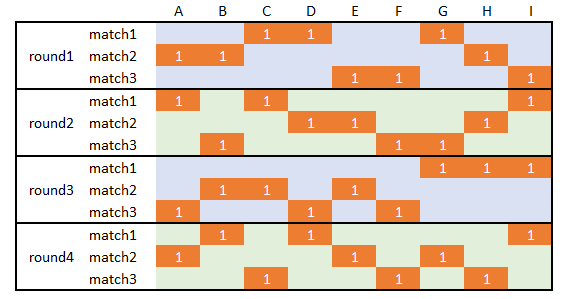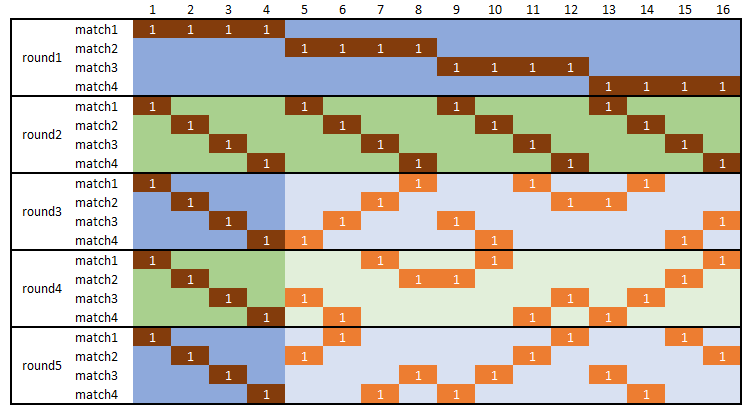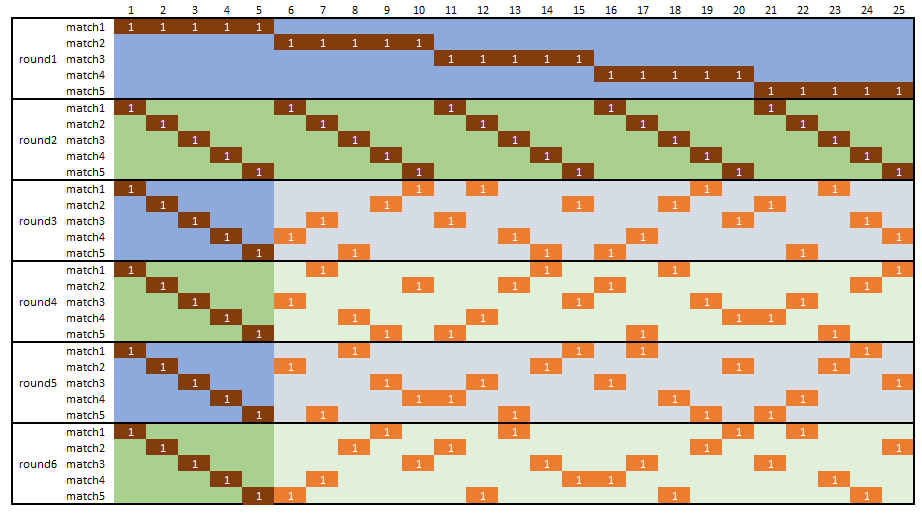## Wednesday, October 3, 2018

### Scheduling and three sided football

#### Design a tournament schedule

In  a question is posed how to design a schedule for a sequence games:

• There are 9 teams,
• we have 4 rounds of 3 matches,
• each match consists of 3 teams,
• a team plays with another team exactly once in the same match.
This problem is related to the social golfer problem [2,3]. Not many games have three teams but there is something called three sided football .Three sided football field 

To find a feasible schedule we use the following sets: \begin{align} & t \in \{A,\dots,I\} && \text{9 teams} \\ & r \in \{\mathit{round}_1,\dots,\mathit{round}_4\} && \text{4 rounds}\\ & m \in \{\mathit{match}_1,\dots,\mathit{match}_3\} && \text{3 matches per round} \end{align} We introduce binary variables $x_{r,m,t} = \begin{cases} 1 & \text{if team t plays in match m in round r} \\ 0 & \text{otherwise}\end{cases}$
A high-level model can look like:

MIQCP feasibility model
$\sum_m x_{r,m,t} = 1 \>\>\forall r,t$Each team plays exactly once in each round
$\sum_t x_{r,m,t} = 3 \>\>\forall r,m$Each match consists of three teams
$\sum_{r,m} x_{r,m,t} x_{r,m,t'} = 1 \>\>\forall t\lt t'$Teams $$t$$ and $$t'$$ play exactly once in the same match. This is a non-convex, quadratic constraint.

There is no objective: we are only looking for a feasible solution. The last equation is the most complicated one. We have $$x_{r,m,t} x_{r,m,t'}=1$$ if teams $$(t,t')$$  are in the same match $$(r,m)$$. Because of symmetry in the product $$x_{r,m,t} x_{r,m,t'}$$ , we only need to check teams $$t\lt t'$$. This saves us a few quadratic constraints.

This model can be solved with a constraint solver or a global (non-convex) MIQCP (Mixed Integer Quadratically Constrained Programming) solver. Of course, with a MIQCP solver we need to add a dummy objective. Global solvers, like Baron, Couenne or Antigone, can solve this easily. The results can look like:Solution
We have marked the cases where $$x_{r,m,t}=1$$. We can check that:

• Every row has three entries (a match has three teams)
• Every column has four entries: one in each round (a team plays once in each round)
• Two teams play in the same match exactly once (e.g. A and B meet in round 1 only)

It is noted that there are many feasible solutions.

#### Making the problem convex, linear

If we want to solve with a convex MIQCP solver (like Cplex or Gurobi) we can replace the quadratic constraint by: $\sum_{r,m} x_{r,m,t} x_{r,m,t'} \le 1 \>\>\forall t\lt t'$

Finally, if we want, we can linearize the quadratic constraint. That would allow us to solve this problem with a straight linear MIP solver. We can write: \begin{align} & y_{r,m,t,t'} \ge x_{r,m,t} + x_{r,m,t'} - 1 && \forall r,m,t\lt t'\\ & \sum_{r,m} y_{r,m,t,t'} \le 1 && \forall t\lt t'\\ & y_{r,m,t,t'} \in [0, 1]\end{align} Again, we need to do this only for $$t\lt t'$$. The MIP model performs better than the quadratic versions if the problems become a bit larger. It is not unusual that linarized models, solved as a MIP, perform better than using a quadratic solver against the original model, even if we have to add a significant number of variables and equations in the process.

There is lots of symmetry in this problem and there are quite a few papers around, mainly from a constraint programming view, that try to deal with this.

#### Fixing

For larger problems there are a few tricks we can apply. For example, we can fix the first two rounds, and let the model only worry about the subsequent rounds. In addition we can fix the first $$m$$ teams in all rounds. The idea is to make the remaining problem smaller so that the solver has an easier job. Fixing also reduces symmetry. With this I could solve very quickly the following instances  (I used the MIP formulation):5 rounds, 4 matches, 16 teams (first 2 rounds, first 4 teams fixed)6 rounds, 5 matches, 25 teams (first 2 rounds, first 5 teams fixed)

The dark areas were fixed and the light area was solved by the MIP solver.

#### Conclusion

With two simple tricks:

• linearization of the quadratic constraint
• fixing a significant part of the problem
we can solve reasonable sized schedule design problems with a good MIP solver quickly.

#### References

1. PHP - Evenly Distribute Teams Into Arrays So That No Combination Repeats, https://stackoverflow.com/questions/52600546/php-evenly-distribute-teams-into-arrays-so-that-no-combination-repeats
2. Markus Triska and Nysret Musliu, An Improved SAT Formulation for the Social Golfer Problem, Annals of Operations Research Vol. 194(1) (2012), pp. 427-438
3. Nicolas Barnier, Pascal Brisset. Solving the Kirkman’s schoolgirl problem in a few seconds. CP 2002, 8th International Conference on Principles and Practice of Constraint Programming, Sep 2002, Ithaca, United States. Springer, 2470, pp 33-41, 2002.
4. Three Sided Football, https://en.wikipedia.org/wiki/Three_sided_football
5. All Match Combinations With Either 4 or 5 Teams Per Match When There are 16 or 25 Teams, https://math.stackexchange.com/questions/2939746/all-match-combinations-with-either-4-or-5-teams-per-match-when-there-are-16-or-2